Hypothesis Tests

PROC TRANSREG has a set of options for testing hypotheses in models with a single dependent variable. The TEST a-option produces an ANOVA table. It tests the null hypothesis that the vector of coefficients for all of the transformations is zero. The SS2 a-option produces a regression table with Type II tests of the contribution of each transformation to the overall model. In some cases, exact tests are provided; in other cases, the tests are approximate, liberal, or conservative.

There are two reasons why it is typically not appropriate to test hypotheses by using the output from PROC TRANSREG as input to other procedures such as the REG procedure. First, PROC REG has no way of determining how many degrees of freedom were used for each transformation. Second, the Type II sums of squares for the tests of the individual regression coefficients are not correct for the transformation regression model because PROC REG, as it evaluates the effect of each variable, cannot change the transformations of the other variables. PROC TRANSREG uses the correct degrees of freedom and sums of squares.

In an ordinary univariate linear model, there is one parameter for each independent variable, including the intercept. In the transformation regression model, many of the variables are used internally in the bases for the transformations. Each basis column has one parameter or scoring coefficient, and each linearly independent column has one model degree of freedom associated with it. Coefficients applied to transformed variables, model coefficients, do not enter into the degrees-of-freedom calculations. They are byproducts of the standardizations and can be absorbed into the transformations by specifying the ADDITIVE a-option. The word parameter is reserved for model and scoring coefficients that have a degree of freedom associated with them.

For expansions, there is one model parameter for each variable created by the expansion (except for all missing CLASS columns and expansions that have an implicit intercept). Each IDENTITY variable has one model parameter. If there are m POINT variables, they expand to m + 1 variables and hence have m + 1 model parameters. For m EPOINT variables, there are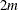model parameters. For m QPOINT variables, there are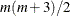model parameters. If a variable with m categories is designated CLASS, there are m – 1 parameters. For BSPLINE and PSPLINE variables of DEGREE=n with NKNOTS=k, there are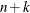parameters. Note that one of the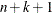BSPLINE columns and one of the m CLASS(variable / ZERO=NONE) columns are not counted due to the implicit intercept.

There are scoring parameters for missing values in nonexcluded observations. Each ordinary missing value (.) has one scoring parameter. Each different special missing value (._ and .A through .Z) within each variable has one scoring parameter. Missing values specified in the UNTIE= and MONOTONE= options follow the rules for UNTIE and MONOTONE transformations, which are described later in this chapter.

For all nonoptimal transformations (LOG, LOGIT, ARSIN, POWER, EXP, RANK, BOXCOX), there is one parameter per variable in addition to any missing value scoring parameters.

For SPLINE, OPSCORE, and LINEAR transformations, the number of scoring parameters is the number of basis columns that are used internally to find the transformations minus 1 for the intercept. The number of scoring parameters for SPLINE variables is the same as the number of model parameters for BSPLINE and PSPLINE variables. If DEGREE=n and NKNOTS=k, there arescoring parameters. The number of scoring parameters for OPSCORE, SMOOTH, and SSPLINE variables is the same as the number of model parameters for CLASS variables. If there are m categories, there are m – 1 scoring parameters. There is one parameter for each LINEAR variable. For SPLINE, OPSCORE, LINEAR, MONOTONE, UNTIE, and MSPLINE transformations, missing value scoring parameters are computed as described previously with the nonoptimal transformations.

The number of scoring parameters for MONOTONE, UNTIE, and MSPLINE transformations is less precise than for SPLINE, OPSCORE, and LINEAR transformations. One way of handling a MONOTONE transformation is to treat it as if it were the same as an OPSCORE transformation. If there are m categories, there are m – 1 potential scoring parameters. However, there are typically fewer than m – 1 unique parameter estimates, since some of those m – 1 scoring parameter estimates might be tied during the optimal scaling to impose the order constraints. Imposing ties on the scoring parameter estimates is equivalent to fitting a model with fewer parameters. So there are two available scoring parameter counts: m – 1 and a smaller number that is determined during the analysis. Using m – 1 as the model degrees of freedom for MONOTONE variables (treating OPSCORE and MONOTONE transformations the same way) is conservative, since the MONOTONE scoring parameter estimates are more restricted than the OPSCORE scoring parameter estimates. Using the smaller count (the number of scoring parameter estimates that are different, minus 1 for the intercept) in the model degrees of freedom is liberal, since the data and the model together are being used to determine the number of parameters. PROC TRANSREG reports tests that use both liberal and conservative degrees of freedom to provide lower and upper bounds on the true p-values.

For the UNTIE transformation, the conservative scoring parameter count is the number of distinct observations, whereas the liberal scoring parameter count is the number of scoring parameter estimates that are different, minus 1 for the intercept. Hence, when you specify UNTIE, conservative tests have zero error degrees of freedom unless there are replicated observations.

For MSPLINE variables of DEGREE=n and NKNOTS=k, the conservative scoring parameter count is, whereas the liberal parameter count is the number of scoring parameter estimates that are different, minus 1 for the intercept. A liberal degrees of freedom of 1 does not necessarily imply a linear transformation. It implies only that n plus k minus the number of ties imposed equals 1. An example of a one-degree-of-freedom nonlinear transformation is a two-piece linear transformation in which the slope of one piece is 0.

The number of scoring parameters is determined during each iteration. After the last iteration, enough information is available for the TEST a-option to produce an ANOVA table that reports the overall fit of the model. If you specify the SS2 a-option, further iterations are necessary to test the contribution of each transformation to the overall model.

The liberal tests do not compensate for overparameterization. For example, requesting a spline transformation with k knots when a linear transformation will suffice results in liberal tests that are actually conservative because too many degrees of freedom are being used for the transformations. To avoid this problem, use as few knots as possible.

In ordinary multiple regression, an F test of the null hypothesis that the coefficient for variable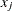is zero can be constructed by comparing two linear models. One model is the full model with all parameters, and the other is a reduced model that has all parameters except the parameter for variable. The difference between the model sum of squares for the full model and the model sum of squares for the reduced model is the Type II sum of squares for the test of the null hypothesis that the coefficient for variableis 0. The numerator of the F test has one degree of freedom. The mean square error for the full model is the denominator of the F test of variable. Note that the estimates of the coefficients for the two models are not usually the same. When variableis removed, the coefficients for the other variables change to compensate for the removal of. In a transformation regression model, the transformations of the other variables must be permitted to change and the numerator degrees of freedom are not always ones. It is not correct to simply let the model coefficients for the transformed variables change and apply the new model coefficients to the old transformations computed with the old scoring parameter estimates. In a transformation regression model, further iteration is needed to test each transformation, because all the scoring parameter estimates for other variables must be permitted to change to test the effect of variable. This can be quite time-consuming for a large model if the SOLVE a-option cannot be used to solve directly for the transformations.